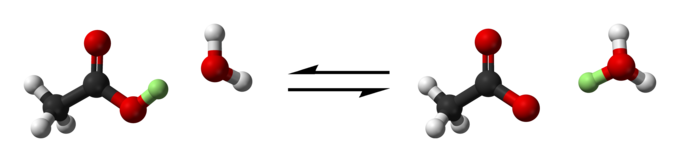## Acid Dissociation Constant (Ka)

#### Learning Objective

• Compare and contrast acid strengths using Ka and pKa values.

#### Key Points

• An acid dissociation constant (Ka) is a quantitative measure of the strength of an acid in solution.
• The dissociation constant is usually written as a quotient of the equilibrium concentrations (in mol/L): $K_a = \frac{[A-][H+]}{[HA]}$ .
• Often times, the Ka value is expressed by using the pKa, which is equal to $-log_{10}(K_a)$ . The larger the value of pKa, the smaller the extent of dissociation.
• A weak acid has a pKa value in the approximate range of -2 to 12 in water. Acids with a pKa value of less than about -2 are said to be strong acids.

#### Terms

• equilibriumThe state of a reaction in which the rates of the forward and reverse reactions are equal.
• dissociationReferring to the process by which a compound breaks into its constituent ions in solution.

The acid dissociation constant (Ka) is a quantitative measure of the strength of an acid in solution. Ka is the equilibrium constant for the following dissociation reaction of an acid in aqueous solution:

$HA(aq) \rightleftharpoons H^+(aq) + A^-(aq)$

In the above reaction, HA (the generic acid), A (the conjugate base of the acid), and H+ (the hydrogen ion or proton) are said to be in equilibrium when their concentrations do not change over time. As with all equilibrium constants, the value of Ka is determined by the concentrations (in mol/L) of each aqueous species at equilibrium. The Ka expression is as follows:

$K_a=\frac{[H^+][A^-]}{[HA]}$

Acid dissociation constants are most often associated with weak acids, or acids that do not completely dissociate in solution. This is because strong acids are presumed to ionize completely in solution and therefore their Ka values are exceedingly large.

## Ka and pKa

Due to the many orders of magnitude spanned by Ka values, a logarithmic measure of the acid dissociation constant is more commonly used in practice. The logarithmic constant (pKa) is equal to -log10(Ka).

The larger the value of pKa, the smaller the extent of dissociation. A weak acid has a pKa value in the approximate range of -2 to 12 in water. Acids with a pKa value of less than about -2 are said to be strong acids. A strong acid is almost completely dissociated in aqueous solution; it is dissociated to the extent that the concentration of the undissociated acid becomes undetectable. pKa values for strong acids can be estimated by theoretical means or by extrapolating from measurements in non-aqueous solvents with a smaller dissociation constant, such as acetonitrile and dimethylsulfoxide.Acetic acid dissociationThe acetic acid partially and reversibly dissociates into acetate and hydrogen ions.

## Example

Acetic acid is a weak acid with an acid dissociation constant $K_a=1.8\times 10^{-5}$ . What is the pKa for acetic acid?

$pK_a=-log(1.8\times 10^{-5})=4.74$